Paul's Online Notes
Home / Calculus I / Review / Trig Equations with Calculators, Part I
Show Mobile Notice Show All Notes Hide All Notes
Mobile Notice
You appear to be on a device with a "narrow" screen width (i.e. you are probably on a mobile phone). Due to the nature of the mathematics on this site it is best views in landscape mode. If your device is not in landscape mode many of the equations will run off the side of your device (should be able to scroll to see them) and some of the menu items will be cut off due to the narrow screen width.

### Section 1.5 : Solving Trig Equations with Calculators, Part I

In the previous section we started solving trig equations. The only problem with the equations we solved in there is that they pretty much all had solutions that came from a handful of “standard” angles and of course there are many equations out there that simply don’t. So, in this section we are going to take a look at some more trig equations, the majority of which will require the use of a calculator to solve (a couple won’t need a calculator).

The fact that we are using calculators in this section does not however mean that the problems in the previous section aren’t important. It is going to be assumed in this section that the basic ideas of solving trig equations are known and that we don’t need to go back over them here. In particular, it is assumed that you can use a unit circle to help you find all answers to the equation (although the process here is a little different as we’ll see) and it is assumed that you can find answers in a given interval. If you are unfamiliar with these ideas you should first go to the previous section and go over those problems.

Before proceeding with the problems we need to go over how our calculators work so that we can get the correct answers. Calculators are great tools but if you don’t know how they work and how to interpret their answers you can get in serious trouble.

First, as already pointed out in previous sections, everything we are going to be doing here will be in radians so make sure that your calculator is set to radians before attempting the problems in this section. Also, we are going to use 4 decimal places of accuracy in the work here. You can use more if you want, but in this class, we’ll always use at least 4 decimal places of accuracy.

Next, and somewhat more importantly, we need to understand how calculators give answers to inverse trig functions. We didn’t cover inverse trig functions in this review, but they are just inverse functions and we have talked a little bit about inverse functions in a review section. The only real difference is that we are now using trig functions. We’ll only be looking at three of them and they are:

$\begin{array}{lcl}{\mbox{Inverse Cosine}} & : & {\cos ^{ - 1}}\left( x \right) = \arccos \left( x \right)\\ {\mbox{Inverse Sine}} & : & {\sin ^{ - 1}}\left( x \right) = \arcsin \left( x \right)\\ {\mbox{Inverse Tangent}} & : & {\tan ^{ - 1}}\left( x \right) = \arctan \left( x \right)\end{array}$

As shown there are two different notations that are commonly used. In these notes we’ll be using the first form since it is a little more compact. Most calculators these days will have buttons on them for these three so make sure that yours does as well.

We now need to deal with how calculators give answers to these. Let’s suppose, for example, that we wanted our calculator to compute $${\cos ^{ - 1}}\left( {{\frac{3}{4}}} \right)$$. First, remember that what the calculator is computing is the angle, let’s say $$x$$, that we would plug into cosine to get a value of $${\frac{3}{4}}$$, or

$x = {\cos ^{ - 1}}\left( {\frac{3}{4}} \right)\hspace{0.5in} \Rightarrow \hspace{0.5in}\cos \left( x \right) = \frac{3}{4}$

So, in other words, when we are using our calculator to compute an inverse trig function we are really solving a simple trig equation.

Having our calculator compute $${\cos ^{ - 1}}\left( {{\frac{3}{4}}} \right)$$ and hence solve $$\cos \left( x \right) = {\frac{3}{4}}$$ gives,

$x = {\cos ^{ - 1}}\left( {\frac{3}{4}} \right) = 0.7227$

From the previous section we know that there should in fact be an infinite number of answers to this including a second angle that is in the interval $$\left[ {0,2\pi } \right]$$. However, our calculator only gave us a single answer. How to determine what the other angles are will be covered in the following examples so we won’t go into detail here about that. We did need to point out however, that the calculators will only give a single answer and that we’re going to have more work to do than just plugging a number into a calculator.

Since we know that there are supposed to be an infinite number of solutions to $$\cos \left( x \right) = {\frac{3}{4}}$$ the next question we should ask then is just how did the calculator decide to return the answer that it did? Why this one and not one of the others? Will it give the same answer every time?

There are rules that determine just what answer the calculator gives when computing inverse trig functions. All calculators will give answers in the following ranges.

$0 \le {\cos ^{ - 1}}\left( x \right) \le \pi \hspace{0.5in} - \frac{\pi }{2} \le {\sin ^{ - 1}}\left( x \right) \le \frac{\pi }{2}\hspace{0.5in} - \frac{\pi }{2} < {\tan ^{ - 1}}\left( x \right) < \frac{\pi }{2}$

If you think back to the unit circle and recall that we think of cosine as the horizontal axis then we can see that we’ll cover all possible values of cosine in the upper half of the circle and this is exactly the range given above for the inverse cosine. Likewise, since we think of sine as the vertical axis in the unit circle we can see that we’ll cover all possible values of sine in the right half of the unit circle and that is the range given above.

For the tangent range look back to the graph of the tangent function itself and we’ll see that one branch of the tangent is covered in the range given above and so that is the range we’ll use for inverse tangent. Note as well that we don’t include the endpoints in the range for inverse tangent since tangent does not exist there.

So, if we can remember these rules we will be able to determine the remaining angle in $$\left[ {0,2\pi } \right]$$ that also works for each solution.

As a final quick topic let’s note that it will, on occasion, be useful to remember the decimal representations of some basic angles. So here they are,

$\frac{\pi }{2} = 1.5708\hspace{0.5in}\pi = 3.1416\hspace{0.5in}\frac{{3\pi }}{2} = 4.7124\hspace{0.5in}2\pi = 6.2832$

Using these we can quickly see that $${\cos ^{ - 1}}\left( {{\frac{3}{4}}} \right)$$ must be in the first quadrant since 0.7227 is between 0 and 1.5708. This will be of great help when we go to determine the remaining angles

So, once again, we can’t stress enough that calculators are great tools that can be of tremendous help to us, but if you don’t understand how they work you will often get the answers to problems wrong.

So, with all that out of the way let’s take a look at our first problem.

Example 1 Solve $$4\cos \left( t \right) = 3$$ on $$\left[-8,10\right]$$.
Show Solution

Okay, the first step here is identical to the problems in the previous section. We first need to isolate the cosine on one side by itself and then use our calculator to get the first answer.

$\cos \left( t \right) = \frac{3}{4}\hspace{0.5in} \Rightarrow \hspace{0.5in}t = {\cos ^{ - 1}}\left( {\frac{3}{4}} \right) = 0.7227$

So, this is the one we were using above in the opening discussion of this section. At the time we mentioned that there were infinite number of answers and that we’d be seeing how to find them later. Well that time is now.

First, let’s take a quick look at a unit circle for this example.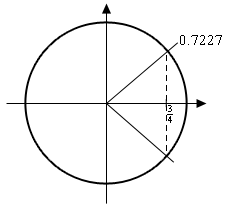The angle that we’ve found is shown on the circle as well as the other angle that we know should also be an answer. Finding this angle here is just as easy as in the previous section. Since the line segment in the first quadrant forms an angle of 0.7227 radians with the positive $$x$$-axis then so does the line segment in the fourth quadrant. This means that we can use either -0.7227 as the second angle or $$2\pi - 0.7227 = 5.5605$$. Which you use depends on which you prefer. We’ll pretty much always use the positive angle to avoid the possibility that we’ll lose the minus sign.

So, all possible solutions, ignoring the interval for a second, are then,

\begin{align*} \begin{aligned}t & = 0.7227 + 2\pi n\\ t & = 5.5605 + 2\pi n\end{aligned} & {\hspace{0.5in}n = 0, \pm 1, \pm 2, \ldots }\end{align*}

Now, all we need to do is plug in values of $$n$$ to determine the angle that are actually in the interval. Here’s the work for that.

$\begin{array}{lclcl}n = - 2 & : & \hspace{0.25in}t = \require{cancel} \xcancel{{ - 11.8437}} & \hspace{0.25in}{\mbox{and}}\hspace{0.25in} & - 7.0059\\ n = - 1 & : & \hspace{0.25in}t = - 5.5605 & \hspace{0.25in}{\mbox{and}}\hspace{0.25in} & - 0.7227\\ n = 0 & : & \hspace{0.25in}t = 0.7227 & \hspace{0.25in}{\mbox{and}}\hspace{0.25in} & 5.5605\\ n = 1 & : & \hspace{0.25in}t = 7.0059 & \hspace{0.25in}{\mbox{and}}\hspace{0.25in} & \xcancel{{11.8437}}\end{array}$

So, the solutions to this equation, in the given interval, are,

$t = - 7.0059,\,\, - 5.5605,\,\,\, - 0.7227,\,\,\,0.7227,\,\,\,5.5605,\,\,\,7.0059$

Note that we had a choice of angles to use for the second angle in the previous example. The choice of angles there will also affect the value(s) of $$n$$ that we’ll need to use to get all the solutions. In the end, regardless of the angle chosen, we’ll get the same list of solutions, but the value(s) of $$n$$ that give the solutions will be different depending on our choice.

Also, in the above example we put in a little more explanation than we’ll show in the remaining examples in this section to remind you how these work.

Example 2 Solve $$- 10\cos \left( {3\,t} \right) = 7$$ on $$\left[-2,5\right]$$.
Show Solution

Okay, let’s first get the inverse cosine portion of this problem taken care of.

$\cos \left( {3\,t} \right) = - \frac{7}{{10}}\hspace{0.5in}\hspace{0.25in} \Rightarrow \hspace{0.5in}3t = {\cos ^{ - 1}}\left( { - \frac{7}{{10}}} \right) = 2.3462$

Don’t forget that we still need the “3”!

Now, let’s look at a quick unit circle for this problem. As we can see the angle 2.3462 radians is in the second quadrant and the other angle that we need is in the third quadrant. We can find this second angle in exactly the same way we did in the previous example. We can use either -2.3462 or we can use $$2\pi - 2.3462 = 3.9370$$. As with the previous example we’ll use the positive choice, but that is purely a matter of preference. You could use the negative if you wanted to.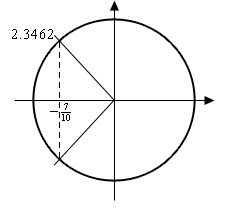So, let’s now finish out the problem. First, let’s acknowledge that the values of 3$$t$$ that we need are,

\begin{align*} \begin{aligned}3t & = 2.3462 + 2\pi n\\ 3t & = 3.9370 + 2\pi n\end{aligned} & {\hspace{0.5in}n = 0, \pm 1, \pm 2, \ldots }\end{align*}

Now, we need to properly deal with the 3, so divide that out to get all the solutions to the trig equation.

\begin{align*} \begin{aligned}t & = 0.7821 + \frac{{2\pi n}}{3}\\ t & = 1.3123 + \frac{{2\pi n}}{3}\end{aligned} & {\hspace{0.5in}n = 0, \pm 1, \pm 2, \ldots }\end{align*}

Finally, we need to get the values in the given interval.

$\begin{array}{lclcl}n = - 2 & : & \hspace{0.25in}t = \require{cancel} \xcancel{{ - 3.4067}} & \hspace{0.25in}{\rm{and}}\hspace{0.25in} & \xcancel{{ - 2.8765}}\\ n = - 1 & : & \hspace{0.25in}t = - 1.3123 & \hspace{0.25in}{\rm{and}}\hspace{0.25in} & - 0.7821\\ n = 0 & : & \hspace{0.25in}t = 0.7821 & \hspace{0.25in}{\rm{and}}\hspace{0.25in} & 1.3123\\ n = 1 & : & \hspace{0.25in}t = 2.8765 & \hspace{0.25in}{\rm{and}}\hspace{0.25in} & 3.4067\\ n = 2 & : & \hspace{0.25in}t = 4.9709 & \hspace{0.25in}{\rm{and}}\hspace{0.25in} & \xcancel{{5.5011}}\end{array}$

The solutions to this equation, in the given interval are then,

$t = - 1.3123,\,\, - 0.7821,\,\,0.7821,\,\,1.3123,\,\,2.8765,\,\,3.4067,\,\,4.9709$

We’ve done a couple of basic problems with cosines, now let’s take a look at how solving equations with sines work.

Example 3 Solve $$\displaystyle 6\sin \left( {\frac{x}{2}} \right) = 1$$ on $$\left[-20,30\right]$$
Show Solution

Let’s first get the calculator work out of the way since that isn’t where the difference comes into play.

$\sin \left( {\frac{x}{2}} \right) = \frac{1}{6}\hspace{0.5in} \Rightarrow \hspace{0.5in}\frac{x}{2} = {\sin ^{ - 1}}\left( {\frac{1}{6}} \right) = 0.1674$

Here’s a unit circle for this example.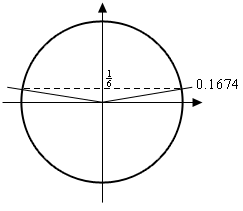To find the second angle in this case we can notice that the line in the first quadrant makes an angle of 0.1674 with the positive $$x$$-axis and so the angle in the second quadrant will then make an angle of 0.1674 with the negative $$x$$-axis. So, if we start at the positive $$x$$-axis we rotate a half revolution and then back off 0.1674. Therefore, the angle that we’re after is then, $$\pi - 0.1674 = 2.9742$$.

Here’s the rest of the solution for this example. We’re going to assume from this point on that you can do this work without much explanation.

\begin{align*}\begin{aligned}\frac{x}{2} & = 0.1674 + 2\pi n\\ & \\ \frac{x}{2} & = 2.9742 + 2\pi n\end{aligned} & {\hspace{0.25in} \Rightarrow \hspace{0.25in}} &\begin{aligned}x & = 0.3348 + 4\pi n\\ x & = 5.9484 + 4\pi n\end{aligned} & {\hspace{0.25in} n = 0, \pm 1, \pm 2, \ldots }\end{align*} $\begin{array}{lclcl}n = - 2 & : & \hspace{0.25in} & x = \require{cancel} \xcancel{{ - 24.7980}} & \hspace{0.25in}{\rm{and}}\hspace{0.25in} & - 19.1844\\ n = - 1 & : & \hspace{0.25in} & x = - 12.2316 & \hspace{0.25in}{\rm{and}}\hspace{0.25in} & - 6.6180\\ n = 0 & : & \hspace{0.25in} & x = 0.3348 & \hspace{0.25in}{\rm{and}}\hspace{0.25in} & 5.9484\\ n = 1 & : & \hspace{0.25in} & x = 12.9012 & \hspace{0.25in}{\rm{and}}\hspace{0.25in} & 18.5148\\ n = 2 & : & \hspace{0.5in} & x = 25.4676 & \hspace{0.25in}{\rm{and}}\hspace{0.25in} & \xcancel{{31.0812}}\end{array}$

The solutions to this equation are then,

$x = - 19.1844,\,\, - 12.2316,\,\, - 6.6180,\,\,0.3348,\,\,5.9484,\,\,12.9012,\,\,18.5148,\,\,25.4676$
Example 4 Solve $$3\sin \left( {5z} \right) = - 2$$ on $$\left[0,1\right]$$.
Show Solution

You should be getting pretty good at these by now, so we won’t be putting much explanation in for this one. Here we go.

$\sin \left( {5z} \right) = - \frac{2}{3}\hspace{0.5in} \Rightarrow \hspace{0.5in}5z = {\sin ^{ - 1}}\left( { - \frac{2}{3}} \right) = - 0.7297$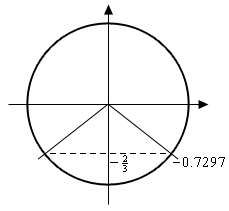Okay, with this one we’re going to do a little more work than with the others. For the first angle we could use the answer our calculator gave us. However, it’s easy to lose minus signs so we’ll instead use $$2\pi - 0.7297 = 5.5535$$. Again, there is no reason to this other than a worry about losing the minus sign in the calculator answer. If you’d like to use the calculator answer you are more than welcome to. For the second angle we’ll note that the lines in the third and fourth quadrant make an angle of 0.7297 with the $$x$$-axis. So, if we start at the positive $$x$$-axis we rotate a half revolution and then add on 0.7297 for the second angle. Therefore, the second angle is $$\pi + 0.7297 = 3.8713$$.

Here’s the rest of the work for this example.

\begin{align*}\begin{aligned}5z & = 5.5535 + 2\pi n\\ 5z & = 3.8713 + 2\pi n\end{aligned} &{\hspace{0.25in} \Rightarrow \hspace{0.25in}} &\begin{aligned}z & = 1.1107 + \frac{{2\pi n}}{5}\\ z & = 0.7743 + \frac{{2\pi n}}{5}\end{aligned} & {\hspace{0.25in} n = 0, \pm 1, \pm 2, \ldots }\end{align*} $\begin{array}{lclcl}n = - 1 & : & \hspace{0.25in} & x = \require{cancel} \xcancel{{ - 0.1460}} & \hspace{0.25in}{\rm{and}}\hspace{0.25in} & \xcancel{{ - 0.4823}}\\ n = 0 & : & \hspace{0.25in} & \xcancel{{x = 1.1107}} & \hspace{0.25in}{\rm{and}}\hspace{0.25in} & 0.7743\end{array}$

So, in this case we get a single solution of 0.7743.

Note that in the previous example we only got a single solution. This happens on occasion so don’t get worried about it. Also, note that it was the second angle that gave this solution and so if we’d just relied on our calculator without worrying about other angles we would not have gotten this solution. Again, it can’t be stressed enough that while calculators are a great tool if we don’t understand how to correctly interpret/use the result we can (and often will) get the solution wrong.

To this point we’ve only worked examples involving sine and cosine. Let’s now work a couple of examples that involve other trig functions to see how they work.

Example 5 Solve $$9\sin \left( {2x} \right) = - 5\cos \left( {2x} \right)$$ on $$\left[-10,0\right]$$.
Show Solution

At first glance this problem seems to be at odds with the sentence preceding the example. However, it really isn’t.

First, when we have more than one trig function in an equation we need a way to get equations that only involve one trig function. There are many ways of doing this that depend on the type of equation we’re starting with. In this case we can simply divide both sides by a cosine and we’ll get a single tangent in the equation. We can now see that this really is an equation that doesn’t involve a sine or a cosine.

So, let’s get started on this example.

$\frac{{\sin \left( {2x} \right)}}{{\cos \left( {2x} \right)}} = \tan \left( {2x} \right) = - \frac{5}{9}\hspace{0.5in} \Rightarrow \hspace{0.5in}2x = {\tan ^{ - 1}}\left( { - \frac{5}{9}} \right) = - 0.5071$

Now, the unit circle doesn’t involve tangents, however we can use it to illustrate the second angle in the range $$\left[ {0,2\pi } \right]$$.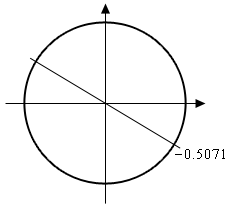The angles that we’re looking for here are those whose quotient of $$\frac{{{\rm{sine}}}}{{{\rm{cosine}}}}$$ is the same. The second angle where we will get the same value of tangent will be exactly opposite of the given point. For this angle the values of sine and cosine are the same except they will have opposite signs. In the quotient however, the difference in signs will cancel out and we’ll get the same value of tangent. So, the second angle will always be the first angle plus $$\pi$$.

Before getting the second angle let’s also note that, like the previous example, we’ll use the $$2\pi - 0.5071 = 5.7761$$ for the first angle. Again, this is only because of a concern about losing track of the minus sign in our calculator answer. We could just as easily do the work with the original angle our calculator gave us.

Now, this is where is seems like we’re just randomly making changes and doing things for no reason. The second angle that we’re going to use is,

$\pi + \left( { - 0.5071} \right) = \pi - 0.5071 = 2.6345$

The fact that we used the calculator answer here seems to contradict the fact that we used a different angle for the first above. The reason for doing this here is to give a second angle that is in the range $$\left[ {0,2\pi } \right]$$. Had we used 5.7761 to find the second angle we’d get $$\pi + 5.7761 = 8.9177$$. This is a perfectly acceptable answer; however, it is larger than $$2\pi$$ (6.2832) and the general rule of thumb is to keep the initial angles as small as possible.

Here are all the solutions to the equation.

\begin{align*}\begin{aligned}2x & = 5.7761 + 2\pi n\\ 2x & = 2.6345 + 2\pi n\end{aligned} & {\hspace{0.25in} \Rightarrow \hspace{0.25in}} & \begin{aligned}x & = 2.8881 + \pi n\\ x & = 1.3173 + \pi n\end{aligned} & {\hspace{0.25in}n = 0, \pm 1, \pm 2, \ldots }\end{align*} $\begin{array}{lclcl}n = - 4 & : & \hspace{0.25in} & x = - 9.6783 & \hspace{0.25in}{\rm{and}}\hspace{0.25in} & \require{cancel} \xcancel{{ - 11.2491}}\\ n = - 3 & : & \hspace{0.25in} & x = - 6.5367 & \hspace{0.25in}{\rm{and}}\hspace{0.25in} & - 8.1075\\ n = - 2 & : & \hspace{0.25in} & x = - 3.3951 & \hspace{0.25in}{\rm{and}}\hspace{0.25in} & - 4.9659\\ n = - 1 & : & \hspace{0.25in} & x = - 0.2535 & \hspace{0.25in}{\rm{and}}\hspace{0.25in} & - 1.8243\\ n = 0 & : & \hspace{0.25in} & \require{cancel} \xcancel{{x = 2.8881}} & \hspace{0.25in}{\rm{and}}\hspace{0.25in} & \xcancel{{1.3173}}\end{array}$

The seven solutions to this equation are then,

$- 0.2535,\,\,\,\, - 1.8243,\,\,\,\, - 3.3951,\,\,\, - 4.9659,\,\,\, - 6.5367,\,\,\,\, - 8.1075,\,\,\,\, - 9.6783$

Note as well that we didn’t need to do the $$n = 0$$ computation since we could see from the given interval that we only wanted negative answers and these would clearly give positive answers.

Before moving on we need to address one issue about the previous example. The solution method used there is not the “standard” solution method. Because the second angle is just $$\pi$$ plus the first and if we added $$\pi$$ onto the second angle we’d be back at the line representing the first angle the more standard solution method is to just add $$\pi n$$ onto the first angle.

If using the calculator answer this would give,

$2x = - 0.5071 + \pi n,\quad n = 0, \pm 1, \pm 2, \ldots$

If using the positive angle corresponding to the calculator answer this would give,

$2x = 5.7761 + \pi n,\quad n = 0, \pm 1, \pm 2, \ldots$

Then dividing by 2 either of the following sets of solutions,

\begin{align*}x & = - 0.2535 + \frac{{\pi n}}{2},\quad n = 0, \pm 1, \pm 2, \ldots \\ x & = 2.8881 + \frac{{\pi n}}{2},\quad n = 0, \pm 1, \pm 2, \ldots \end{align*}

Either of these sets of solutions is identical to the set of solutions we got in the example (we’ll leave it to you to plug in some $$n$$’s and verify that). So, why did we not use the method in the previous example? Simple. The method in the previous example more closely mirrors the solution method for cosine and sine (i.e. they both, generally, give two sets of angles) and so for students that aren’t comfortable with solving trig equations this gives a “consistent” solution method.

Many calculators today can only do inverse sine, inverse cosine, and inverse tangent. So, let’s see an example that uses one of the other trig functions.

Example 6 Solve $$7\sec \left( {3t} \right) = - 10$$.
Show Solution

We’ll start this one in exactly the same way we’ve done all the others.

$\sec \left( {3t} \right) = - \frac{{10}}{7}\hspace{0.5in}\hspace{0.25in} \Rightarrow \hspace{0.5in}3t = {\sec ^{ - 1}}\left( { - \frac{{10}}{7}} \right)$

Now we reach the problem. As noted above, many calculators can’t handle inverse secant so we’re going to need a different solution method for this one. To finish the solution here we’ll simply recall the definition of secant in terms of cosine and convert this into an equation involving cosine instead and we already know how to solve those kinds of trig equations.

$\frac{1}{{\cos \left( {3t} \right)}} = \sec \left( {3t} \right) = - \frac{{10}}{7}\hspace{0.5in} \Rightarrow \hspace{0.5in}\cos \left( {3t} \right) = - \frac{7}{{10}}$

Now, we solved this equation in the second example above so we won’t redo our work here. The solution is,

\begin{align*}\begin{aligned}t & = 0.7821 + \frac{{2\pi n}}{3}\\ t & = 1.3123 + \frac{{2\pi n}}{3}\end{aligned} &{\hspace{0.25in}n = 0, \pm 1, \pm 2, \ldots }\end{align*}

We weren’t given an interval in this problem so here is nothing else to do here.

For the remainder of the examples in this section we’re not going to be finding solutions in an interval to save some space. If you followed the work from the first few examples in which we were given intervals you should be able to do any of the remaining examples if given an interval.

Also, we will no longer be including sketches of unit circles in the remaining solutions. We are going to assume that you can use the above sketches as guides for sketching unit circles to verify the claims in the following examples.

The next three examples don’t require a calculator but are important enough or cause enough problems for students to include in this section in case you run across them and haven’t seen them anywhere else.

Example 7 Solve $$\cos \left( {4\theta } \right) = - 1$$.
Show Solution

There really isn’t too much to do with this problem. It is, however, different from all the others done to this point. All the others done to this point have had two angles in the interval $$\left[ {0,2\pi } \right]$$ that were solutions to the equation. This only has one. If you aren’t sure you believe this sketch a quick unit circle and you’ll see that in fact there is only one angle for which cosine is -1.

Here is the solution to this equation.

$4\theta = \pi + 2\pi n\hspace{0.25in} \Rightarrow \hspace{0.25in}\theta = \frac{\pi }{4} + \frac{{\pi n}}{2}\hspace{0.25in}n = 0, \pm 1, \pm 2, \ldots$
Example 8 Solve $$\displaystyle \sin \left( {\frac{\alpha }{7}} \right) = 0$$.
Show Solution

Again, not much to this problem. Using a unit circle it isn’t too hard to see that the solutions to this equation are,

\begin{align*}\begin{aligned}\frac{\alpha }{7} & = 0 + 2\pi n\\ & \\ \frac{\alpha }{7} & = \pi + 2\pi n\end{aligned} & {\hspace{0.25in} \Rightarrow \hspace{0.25in}} & \begin{aligned}\alpha & = 14\pi n\\ \alpha & = 7\pi + 14\pi n\end{aligned} & \hspace{0.25in}{n = 0, \pm 1, \pm 2, \ldots }\end{align*}

This next example has an important point that needs to be understood when solving some trig equations.

Example 9 Solve $$\sin \left( {3t} \right) = 2$$.
Show Solution

This example is designed to remind you of certain properties about sine and cosine. Recall that $$- 1 \le \sin \left( \theta \right) \le 1$$ and $$- 1 \le \cos \left( \theta \right) \le 1$$. Therefore, since sine will never be greater that 1 it definitely can’t be 2. So, THERE ARE NO SOLUTIONS to this equation!

It is important to remember that not all trig equations will have solutions.

Because this document is also being prepared for viewing on the web we’re going to split this section in two in order to keep the page size (and hence load time in a browser) to a minimum. In the next section we’re going to take a look at some slightly more “complicated” equations. Although, as you’ll see, they aren’t as complicated as they may at first seem.